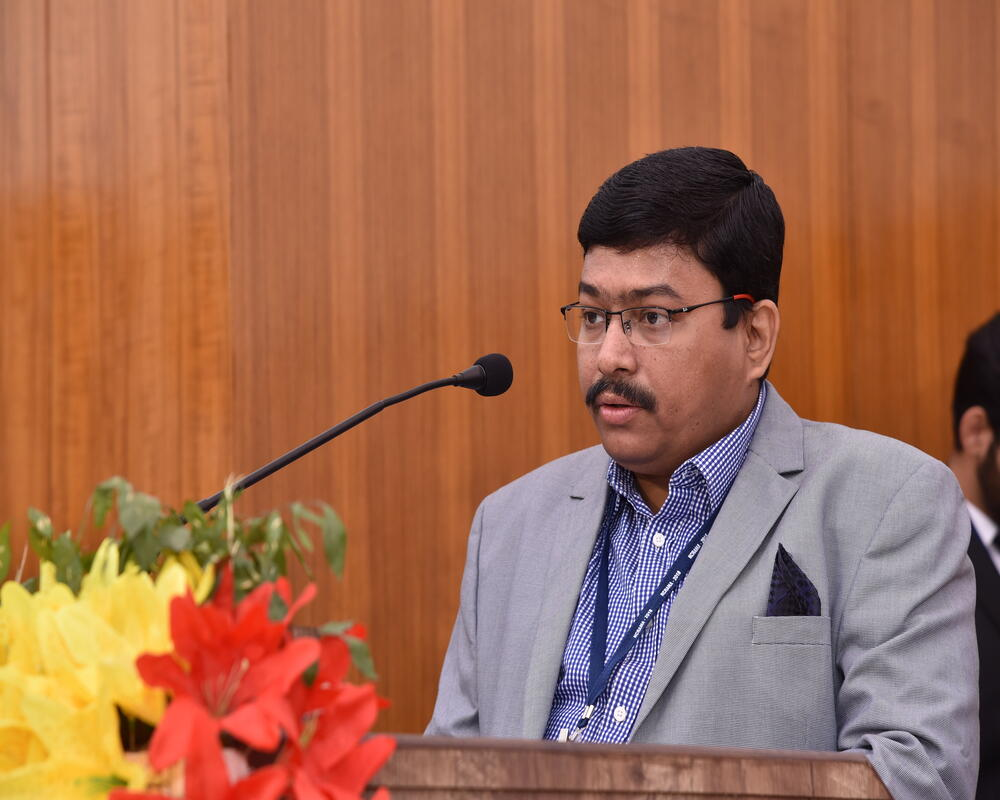|   |   |    WebMail |    NITRis
Call Us +91661 246 2020
Mail Us registrar[at]nitrkl.ac.in#### MathematicsThere is a quote by the famous German mathematician Karl Friedrich Gauss “Mathematics is the queen of all sciences”. He was quite justified because without mathematics, existence of any kind of science is impossible. Mathematics expresses itself everywhere, in almost every facet of life - in nature all around us, and in the technologies in our hands. Mathematics is the language of science and engineering - describing our understanding of all that we observe.

The Department of Mathematics, NIT Rourkela is specialized in creating human resources in the area of Algebraic topology, Functional analysis, Artificial intelligence, Boundary value problems, Category theory, Complex analysis, Differential equations, Differentiable manifolds, Fluid dynamics, Fuzzy set and topology, Fractional calculus, Generic algorithm, Lee algebra, Magneto-hydro dynamics, Mathematical modeling and simulation, Mathematical physics, Number theory, Numerical analysis, Non-linear optimization, Mathematical programming, Statistical inference, Stochastic Processes, Vibration Problems and Wavelet Transforms.### Prof. Santanu Saha Ray

Professor
0661-2462710 (HOD)
0661-2462709 (Off)
hod-ma[at]nitrkl.ac.in